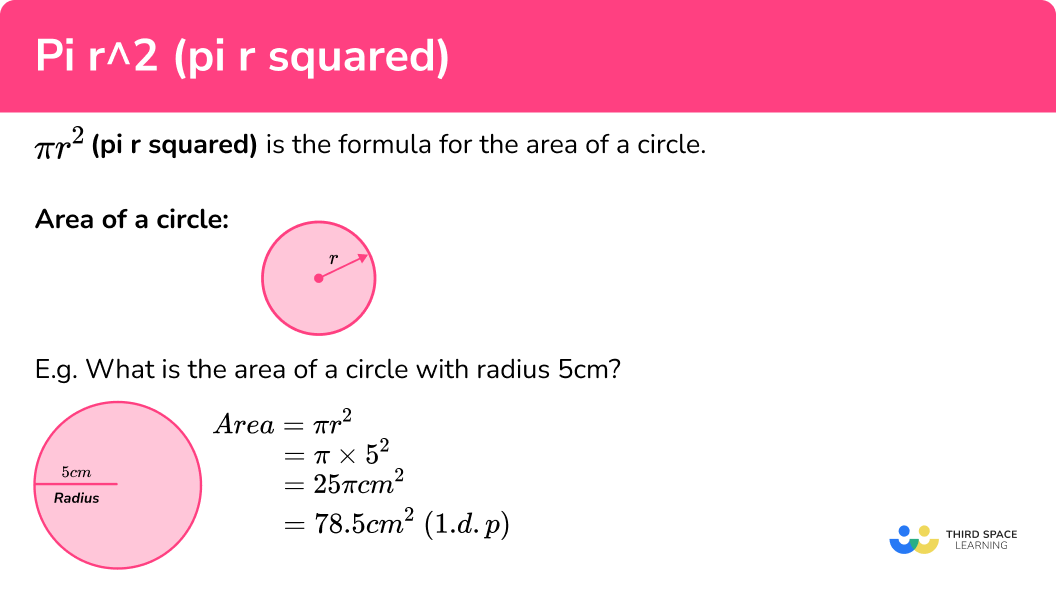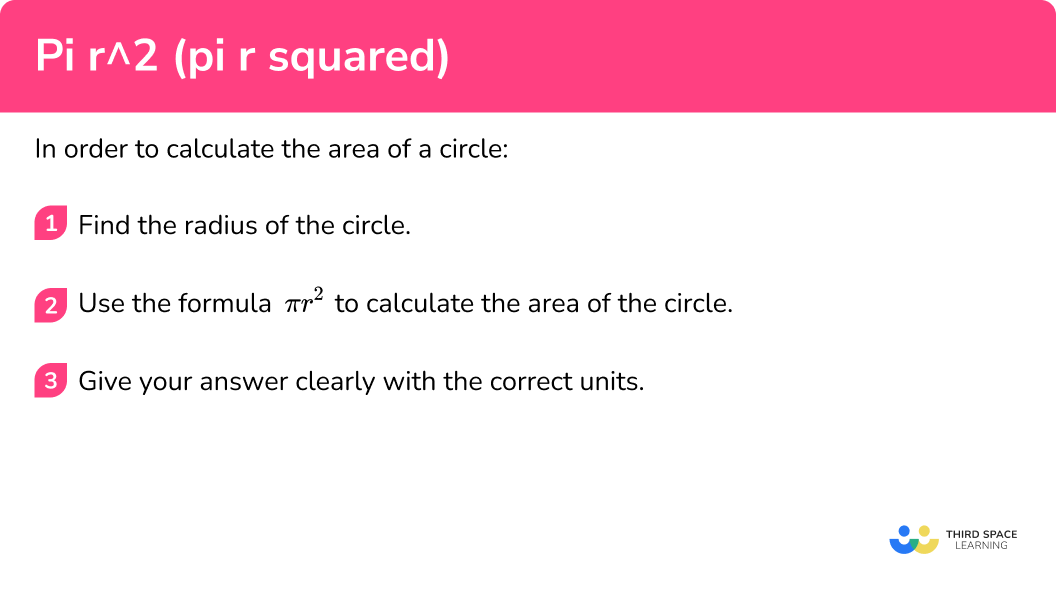# 𝝅r2 (Pi R Squared)

Here we will learn about using the formula \pi r^2 (pi r squared) to calculate the area of a circle given the radius, diameter or the circumference.

There are also worksheets based on Edexcel, AQA and OCR exam questions, along with further guidance on where to go next if you’re still stuck.

## What is pi r squared?

Pi r squared is the formula for the area of a circle.

This is because there is a specific relationship between the radius ( r ) of a circle and its area.

Area of a circle formula:

\pi \times r\times r

we usually simplify this to

Area= \pi r^2

E.g.

What is the area of a circle with radius 5cm ? Give your answer to one decimal place.

Squaring the radius of 5 gives 25. Then multiply pi by this value to work out the area of the circle.

\begin{aligned} \text { Area } &=\pi r^{2} \\\\ &=\pi \times 5^{2} \\\\ &=25 \pi \mathrm{cm}^{2} \\\\ &=78.5 \mathrm{~cm}^{2}(1 . \mathrm{d} . \mathrm{p}) \end{aligned}

### What is pi r squared?### What is pi?

\pi (pronounced pi) is a greek letter that represents the ratio of the circumference of a circle to its diameter.

For all circles if you divide the length of the circumference by the length of the diameter you get the value \pi .

Note: \pi is an irrational number which means it cannot be written as a fraction. It is a non recurring decimal and has an approximate value of 3.1415…

In GCSE you should use the \pi button on your Casio calculator when working with .

To get this you need to press [SHIFT][ \times 10^x ], see below.

Sometimes the question may ask you to give the answer ‘in terms of \pi ’.

E.g.

3 \times \pi = 3 \pi (this is an answer in terms of pi)

5 \times \pi = 5 \pi (this is an answer in terms of pi)

17 \times \pi = 53.407... (this is an answer not in terms of pi)

## How to use pi r squared

In order to calculate the area of a circle:

1. Find the radius of the circle.
2. Use the formula \bf{\pi r^2} to calculate the area of the circle.

### How to use pi r squared## Pi r squared examples

### Example 1: calculating the area of the circle given the radius

1. Find the radius of the circle.

The radius is given in the question

2Use the formula \bf{\pi r^2} to calculate the area of the circle.

\begin{aligned} &\pi r^2 \\\\ &\pi \times r\times r \\\\ &\pi \times7\times 7 \\\\ &49\pi \\\\ &=153.93904... \end{aligned}

153.94

153.94cm^2

### Example 2: calculating the area of the circle given the diameter

The question gives you the diameter which is twice the radius.

Diameter = 20mm

\begin{aligned} &\pi r^2 \\\\ &\pi \times r\times r \\\\ &\pi \times10\times 10 \\\\ &100\pi \\\\ &=314.159... \end{aligned}

314.2

314.2mm^2

### Example 3: calculating the area of the circle, given the radius, answer in terms of 𝝅?

The radius is given in the question

\begin{aligned} &\pi r^2 \\\\ &\pi \times r\times r \\\\ &\pi \times18\times 18 \\\\ &324\pi \end{aligned}

Remember the question asks you to give your answer to ‘in terms of \pi ’. Therefore you leave the answer in the form 324 \pi

324\pi \; m^2

### Example 4: calculating the area of the circle given the diameter

The question gives you the diameter which is twice the radius.

Diameter = 210km

\begin{aligned} &\pi r^2 \\\\ &\pi \times r\times r \\\\ &\pi \times105\times 105 \\\\ &11025\pi \end{aligned}

Remember the question asks you to give your answer to ‘in terms of \pi . Therefore you leave the answer in the form 11025 \pi

11025\pi \; km^2

### Example 5: calculating the area of the circle given the circumference of a circle

A circle has a circumference of 12cm.

Calculate its area.

The question gives you the circumference of the circle which is equal to 2\pi r

This means you can find the radius of the circle from the circumference, see below:

Circumference = 2\pi r

Circumference = 12

\begin{aligned} &12 = 2\pi r \quad \quad \quad \text{ Divide both sides by } 2\pi \\\\ &\frac{12}{2\pi}= r \\\\ &1.9099=r \end{aligned}

Notice how r has been rounded to more decimal places than the question requires so you do not cause a rounding error.

\begin{aligned} &\pi r^2 \\\\ &\pi \times r\times r \\\\ &\pi \times1.9099\times 1.9099 \\\\ &11.4596 \end{aligned}

11.46

11.46cm^2

### Example 6: calculating the area of a semicircle given the diameter

The question gives you the diameter which is twice the radius.

Diameter = 200m

\begin{aligned} &\pi r^2 \\\\ &\pi \times r\times r \\\\ &\pi \times 100\times 100 \\\\ &10000\pi \end{aligned}

Remember the question asks you to give your answer to ‘in terms of \pi . Therefore you leave the answer in the form 10000 \pi

10000\pi

This is the area of a whole circle with a diameter of 200m. You only want the area of a semicircle.

A semicircle has half the area of a full circle so you need to divide your answer by two. Remember to keep it in terms of \pi .

\begin{aligned} &10000 \pi \div 2 \\\\ &5000\pi \end{aligned}

Remember the question asks you to give your answer to ‘in terms of \pi . Therefore you leave the answer in the form 5000 \pi

5000 \pi \; m^2

### Common misconceptions

You must have the radius to find the area of a circle from the formula.

A question may not give you the radius directly and so we need to use the information given to find the radius first.

• Not including the correct units

When working with area you must always give the correct units squared

E.g.

cm^2, \; m^2, \; km^2 etc.

• Not rounding correctly

These questions often involve rounding. You must only round at the end of the question and to the specified number of decimal places.

• Not giving answer in terms of \pi

Sometimes the question may ask you to give the answer ‘in terms of \pi ’. This means you do not give the numerical answer that is produced when you multiply it by \pi .

E.g.

6 \times \pi = 6\pi (this is an answer in terms of pi)

6 \times \pi = 18.8495592… (this answer is not in terms of pi)

• Misuse of calculator

Ensure you know how to correctly use the \pi button on your calculator .

We can also measure angles in radians, however at GCSE we will always measure angles in degrees.

### Practice pi r squared questions

1. A circle has a diameter of 12cm . What is the radius of the circle

6cm3cm12cm6\pi cmThe diameter of the circle is twice the size of the radius. Therefore to find the radius you can divide the diameter by 2.

6cm \div 2 = 3cm

2. What is the formula for the area of a circle

\pi d2\pi r\pi r^2\pi r\pi \times r\times r

we usually simplify this to

\text{Area } =\pi r^2

3. A circle has a radius of 2cm. What is its area to 1 decimal place?

4\pi cm^24\pi cm12.6cm12.6 cm^2\text{Area of a circle } =\pi r^2

\pi \times 2 \times 2 is equal to 12.566...

This answer is correctly rounded to 1 decimal place and has the correct units

4. A circle has a radius of 9cm. What is its area in terms of \pi ?

9\pi cm^2324\pi cm^218\pi cm^281\pi cm^2\text{Area of a circle } =\pi r^2

\pi \times 9 \times 9

81 \pi

This answer is correctly given in terms of \pi

5. A circle has a diameter of 8cm. What is its area in terms of \pi ?

16\pi cm^264\pi cm^28\pi cm^22\pi cm^28cm = diameter

\text{Area of a circle } = \pi r^2

\pi \times 4 \times 4

16 \pi

This answer is correctly given in terms of \pi

6. A circle has a radius of 10cm. What is its area to the nearest whole number?

314 cm^2314.1 cm^2314.159 cm^2100\pi cm^2\text{Area of a circle } = \pi r^2

\pi \times 10 \times 10 is equal to 314.1592654...

This answer is correctly rounded to 1 decimal place and has the correct units

### Pi r squared GCSE questions

1. The radius of a circle is 4.5 cm

Work out the area of the circle

(3 marks)

\pi \times 4.5 \times 4.5    or    63.617…

(1)

63.6

(1)

cm^2

(1)

2. The radius of a circle is 19.1 m

Work out the area of the circle

(3 marks)

\pi \times19.1 \times 19.1    or    1146.0844…

(1)

1146.08

(1)

m^2

(1)

3. The diameter of a circle is 18cm

Work out the area of the circle

(3 marks)

\pi \times9\times 9

(1)

81\pi

(1)

cm^2

(1)

4. The area of a circle is 49 \pi \; cm^2

Calculate the radius of the circle

(3 marks)

49\pi = \pi r^2

(1)

49= r^2

(1)

r=7

(1)

## Learning checklist

You have now learned how to:

• Identify and apply circle definitions and properties, including: centre, radius, chord, diameter, circumference
• Know and apply the formulae \pi r^2
• Give answers in terms of \pi
• Calculate area of 2 D shapes including circles and semicircles

## Still stuck?

Prepare your KS4 students for maths GCSEs success with Third Space Learning. Weekly online one to one GCSE maths revision lessons delivered by expert maths tutors.

Find out more about our GCSE maths tuition programme.